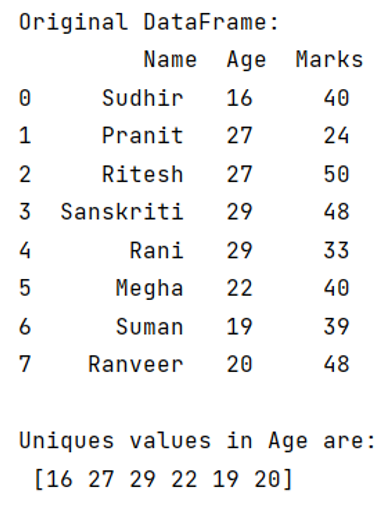# How to count unique values per groups with Pandas?

Given a DataFrame, we have to count unique values per groups.
Submitted by Pranit Sharma, on May 13, 2022

Columns are the different fields that contain their particular values when we create a DataFrame. We can perform certain operations on both rows & column values. In this article, we are going to learn how to count unique values in a column.

Unique values are those values that only occur once.

For this purpose, we are going to use a pandas.Series.unique() method inside pandas. This method will remove all the occurrences except the first occurrence,

## pandas.Series.unique() Method

This method selects the specified column passed as a parameter inside it and returns the unique values inside it.

Syntax:

```Series.unique()

# or
DataFrame['col_name'].unique()
```

To work with pandas, we need to import pandas package first, below is the syntax:

```import pandas as pd
```

Let us understand with the help of an example:

```# Importing pandas package
import pandas as pd

# Create a dictionary for the dataframe
dict = {
'Name': ['Sudhir', 'Pranit', 'Ritesh','Sanskriti', 'Rani','Megha','Suman','Ranveer'],
'Age': [16, 27, 27, 29, 29,22,19,20],
'Marks': [40, 24, 50, 48, 33,40,39,48]
}

# Converting Dictionary to Pandas Dataframe
df = pd.DataFrame(dict)

# Display original DataFrame
print("Original DataFrame:\n",df,"\n")

# Selecting column Age and finding unique values
result = df['Age'].unique()

# Display result
print("Unique values in Age are:\n",result)
```

Output:Preparation